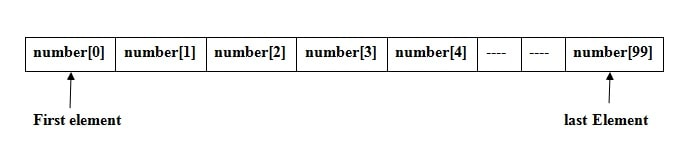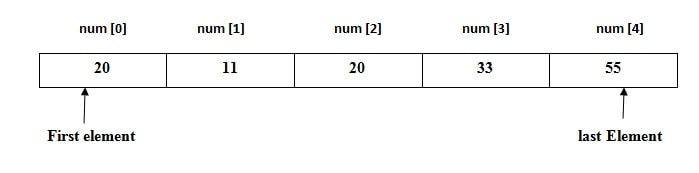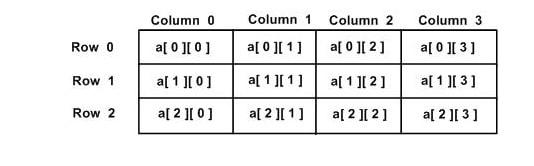# Single and Multidimensional Arrays in C++

Arrays is a kind of data structure that can store a elements of the same type.

Arrays stores the elements in a contiguous memory locations.

Array is a collection of variables of the same type.

For example: we want to declare 100 integer variable

Instead of declaring 100 individual variables, such as

int number0, number1,number2,number3, … …., number99;

you declare one integer array variable such as

int number;

here number is an integer array of size 100, means this array can store 100 integer value.

Array indexing starts from index 0 to n-1.

Means first integer number store in array number, second integer number store in array number, third integer number store in array number, and so on. Here 100th  number store in array numbers.Array In C++

## Declaring Arrays

Syntax of declare an array in C:

Data_type  arrayName [ arraySize ];

This is called a single-dimensional array.

The arraySize must be an integer constant greater than zero.

datatype can be any valid C data type.

Example: write a program to create integer array and store 5 integer number and print.

OutputElement Position in Array

Description : In the above program,   “int  num” array can store 5 element in a contiguous memory locations from index 0 to 4.

Example: Write a program to take 5 number from user and store integer number in integer array and print.

Output

## Another way to Initialize Array

We can also initialize array by this way:

int num = { 54 , 4,  13,  2,  17 };

above integer array num, stores five numbers. Where num store 54, num store 4….. , & num store 17.

Output

## Multi-dimensional Arrays

C++  programming language also support multidimensional arrays.

Syntax of multidimensional array declaration:

Data_type    array_name[size1][size2]…[sizeN];

For example, if we want to creates a three dimensional integer array −

int  num;

### Two-dimensional Arrays

The simplest form of multidimensional array is the two-dimensional array.

If we want to declare a two dimensional integer array of size [x][y] ( where x is a  number of rows and y is a number of columns ) you would write something as follows −

int  a;

In the above line we have created two-dimensional integer array ”a” with 3 rows and 4 columns.Two Dimensional Array

Initializing Two-Dimensional Arrays

In a C++ programming language  Multidimensional arrays may be initialized by specifying bracketed values for each row.

For example we want to create an integer array with 3 rows and each row has 4 columns.

Above the nested braces indicates the intended rows.

The following initialization is equivalent to the previous example −

int a = {1,2,3,4,5,6,7,8,9,10,11,12};

which indicate the nested braces for intended row, are optional.

Accessing Elements of Two-Dimensional Array

Create a two-dimensional array of size 3*3 and  nested loop is used to handle a two-dimensional array −

Output

Example: Write a program to create 3*3 matrix and take all elements of matrix as an input from user and print it.

Output

### CPP Program to Addition of Two Matrix

Example: Write a program to add two matrix.

Firstly, ask from user order of matrix( number of rows and column).

Then take the elements of matrix from user as a input and print the resultant matrix

For example, if a user input order as 3,3, i.e., three rows and three columns and

First matrix

1          2          3
4          5          6
7          8          9

Second matrix:

9          8          7
6          5          4
3          2          1

then the output of the program (addition of the two matrices) is:

10        10        10
10        10        10
10        10        10

Matrix addition program in C++

Output

### Matrix multiplication in CPP

Example: Write a program to multiply two matrix. Firstly, ask from user order of matrix( number of rows and column). Then take the elements of matrix from user as a input and print the resultant matrix.

Read More

Functions in C++

Categories C++